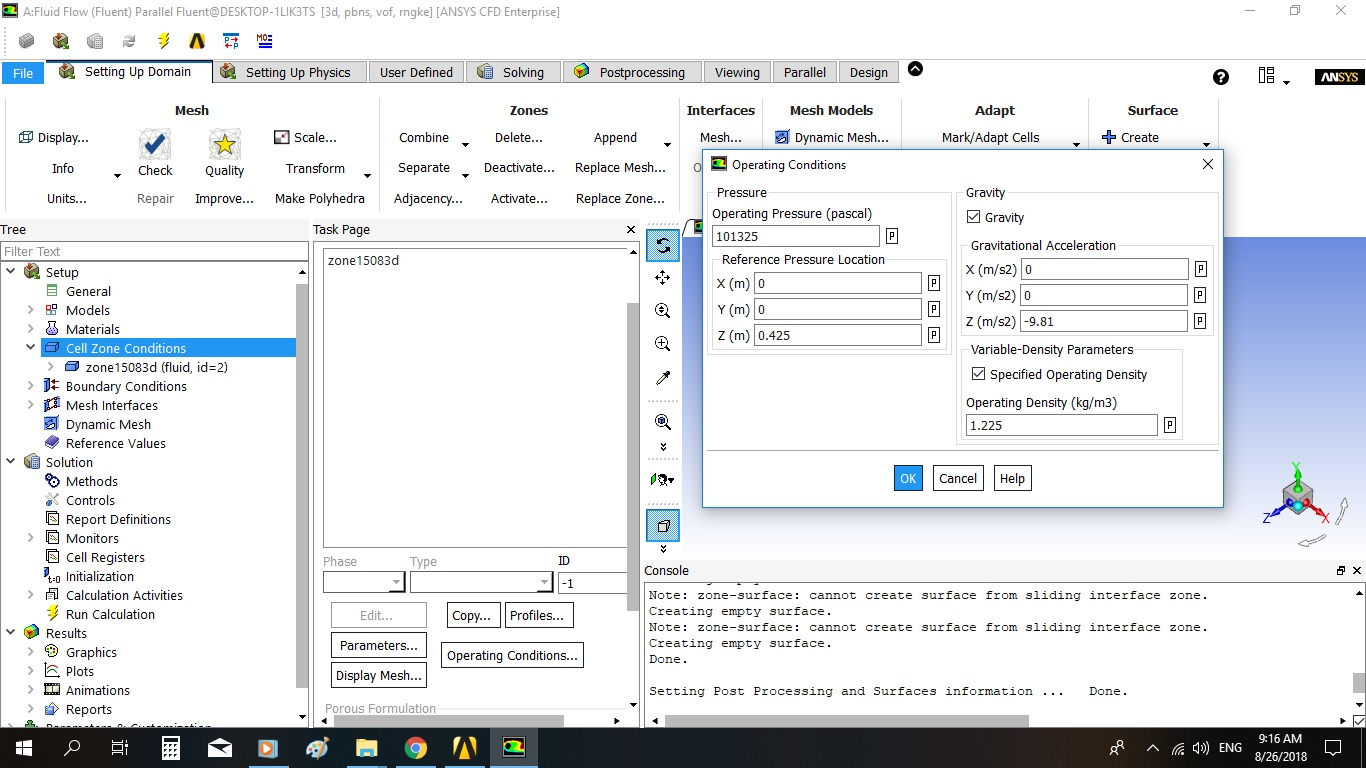Walaa
Subscriber

It happens again, this time i set the following;

volume of fraction cutoff = 0

volume of fraction coaurant number = .25

time scale factor =2

and my geometry height in z direction is .5, the water height is .0296, and i set reference pressure location = .425